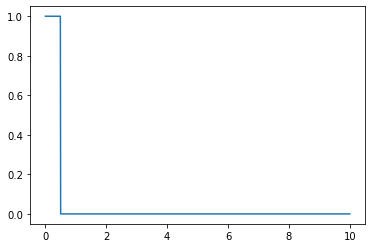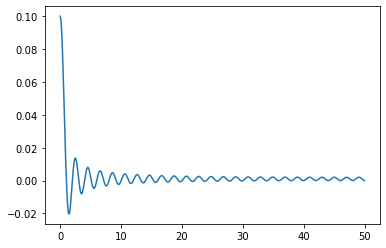# Fourier¶

In :
import numpy as np
import matplotlib.pyplot as plt

N = 1000
T = 0.01
x = np.linspace(0.0, N*T, N)
y = np.where(abs(x)<=0.5, 1, 0) # Rectangular function
plt.plot(x, y);In :
yf = np.fft.fft(y)
xf = np.linspace(0.0, 1.0/(2.0*T), N//2)

plt.plot(xf, 2.0/N * yf[:N//2].real);# Write XLSX¶

In :
# You could read a real CSV file instead
csv_lines = ['a;b;c;d\n', '1;2;3;4\n', '5;6;7;8\n']

In :
import xlsxwriter

workbook  = xlsxwriter.Workbook('filename.xlsx')

i = 0 # line number
for line in csv_lines:
date, time, gh, ta = line.replace('\n', '').split(';')
worksheet.write(i, 0, date)
worksheet.write(i, 1, time)
worksheet.write(i, 2, gh)
worksheet.write(i, 3, ta)
i += 1

workbook.close()


In :
import pandas as pd

In :
pd.read_excel('filename.xlsx')

Out:
a b c d
0 1 2 3 4
1 5 6 7 8

# Remove file¶

BE VERY CAREFUL!

In [ ]:
import os

In [ ]:
os.remove('filename.xlsx')